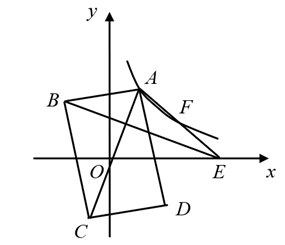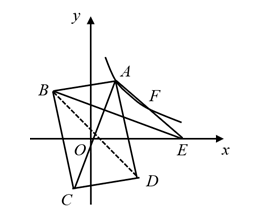$\text{A.}$ 6 $\text{B.}$ 12 $\text{C.}$ 18 $\text{D.}$ 24

B

#### 解析：$\because$ 四边形 $\mathrm{ABCD}$ 为矩形, $\mathrm{O}$ 为对角线,
$$\therefore \mathrm{AO}=\mathrm{OD} \text {, }$$
$$\therefore \angle \mathrm{ODA}=\angle \mathrm{OAD} \text {, }$$

\begin{aligned} & \therefore \angle \mathrm{OAD}=\angle \mathrm{EAD}, \\ & \therefore \angle \mathrm{EAD}=\angle \mathrm{ODA}, \\ & \therefore \mathrm{OB} / / \mathrm{AE} \\ & \because \mathrm{S}_{\triangle \mathrm{ABE}}=18 \\ & \therefore \mathrm{S}_{\triangle \mathrm{OAE}}=18 \end{aligned}

$$\because \mathrm{AF}=\mathrm{EF} \text {, }$$
$\therefore \mathrm{F}$ 点的纵坐标为 $\frac{k}{2 a}$,

$\therefore \mathrm{E}$ 点的坐标为 $(3 \mathrm{a}, 0)$,
$\mathrm{S}_{\triangle \mathrm{OAE}}=\frac{1}{2} \times 3 \mathrm{a} \times \frac{k}{a}=18$,

①点击 首页查看更多试卷和试题 , 点击查看 本题所在试卷